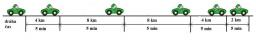# Passenger 2688

The distance between P and T is 89 km. At 8:00 AM, a lorry set off from T at a speed of 28 km/h, and at 8:45 AM, a passenger car set off against it from P at 52 km/h. When and at what distance will they compete?

Result

t = 9:36 hs =  44.8 km

### Step-by-step explanation:

s = 28(t-8.00)
s+52(t-(8+45/60))=89

s = 28·(t-8.00)
s+52·(t-(8+45/60))=89

s-28t = -224
60s+3120t = 32640

Pivot: Row 1 ↔ Row 2
60s+3120t = 32640
s-28t = -224

Row 2 - 1/60 · Row 1 → Row 2
60s+3120t = 32640
-80t = -768

t = -768/-80 = 9.6
s = 32640-3120t/60 = 32640-3120 · 9.6/60 = 44.8

s = 224/5 = 44.8
t = 48/5 = 9.6

Our linear equations calculator calculates it.Did you find an error or inaccuracy? Feel free to write us. Thank you!

Tips for related online calculators
Do you have a system of equations and looking for calculator system of linear equations?
Do you want to convert velocity (speed) units?
Do you want to convert time units like minutes to seconds?